Courses

# Test: Butterworth Filters Design - 1

## 10 Questions MCQ Test Digital Signal Processing | Test: Butterworth Filters Design - 1

Description
This mock test of Test: Butterworth Filters Design - 1 for Electrical Engineering (EE) helps you for every Electrical Engineering (EE) entrance exam. This contains 10 Multiple Choice Questions for Electrical Engineering (EE) Test: Butterworth Filters Design - 1 (mcq) to study with solutions a complete question bank. The solved questions answers in this Test: Butterworth Filters Design - 1 quiz give you a good mix of easy questions and tough questions. Electrical Engineering (EE) students definitely take this Test: Butterworth Filters Design - 1 exercise for a better result in the exam. You can find other Test: Butterworth Filters Design - 1 extra questions, long questions & short questions for Electrical Engineering (EE) on EduRev as well by searching above.
QUESTION: 1

### Which of the following is a frequency domain specification?

Solution:

Explanation: We are required to design a low pass Butterworth filter to meet the following frequency domain specifications.
KP ≤ 20 log|H(jΩ)| ≤ 0
and 20 log|H(jΩ)| ≤ KS.

QUESTION: 2

### What is the value of gain at the pass band frequency, i.e., what is the value of KP?

Solution:

Explanation: We know that the formula for gain is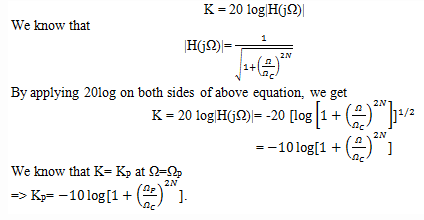QUESTION: 3

### What is the value of gain at the stop band frequency, i.e., what is the value of KS?

Solution:

Explanation: We know that the formula for gain is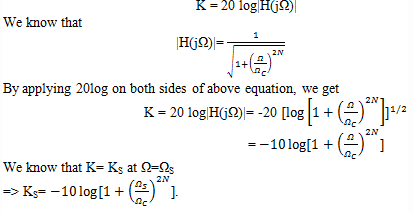QUESTION: 4

Which of the following equation is True?

Solution:

Explanation: We know that,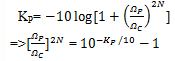QUESTION: 5

Which of the following equation is True?

Solution:

Explanation: We know that,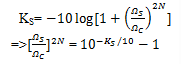QUESTION: 6

What is the order N of the low pass Butterworth filter in terms of KP and KS?

Solution:

Explanation: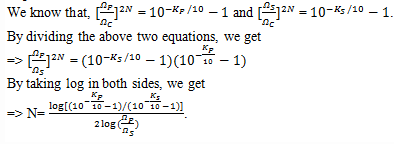QUESTION: 7

What is the expression for cutoff frequency in terms of pass band gain?

Solution:

Explanation: We know that,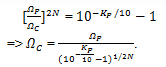QUESTION: 8

What is the expression for cutoff frequency in terms of stop band gain?

Solution:

Explanation: We know that,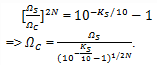QUESTION: 9

The cutoff frequency of the low pass Butterworth filter is the arithmetic mean of the two cutoff frequencies as found above.

Solution:

Explanation: The arithmetic mean of the two cutoff frequencies as found above is the final cutoff frequency of the low pass Butterworth filter.

QUESTION: 10

What is the lowest order of the Butterworth filter with a pass band gain KP= -1 dB at ΩP= 4 rad/sec and stop band attenuation greater than or equal to 20dB at ΩS= 8 rad/sec?

Solution:

Explanation: We know that the equation for the order of the Butterworth filter is given as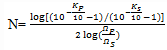From the given question,
KP= -1 dB, ΩP= 4 rad/sec, KS= -20 dB and ΩS= 8 rad/sec
Upon substituting the values in the above equation, we get
N=4.289
Rounding off to the next largest integer, we get N=5.